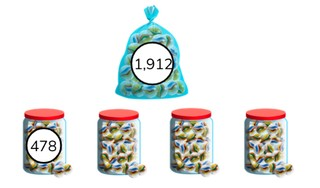Dividing numbers greater than 1,000

# Dividing numbers greater than 1,000

The students learn to divide numbers greater than 1,000.8,000 schools use Gynzy92,000 teachers use Gynzy1,600,000 students use Gynzy

## General

The students learn to divide number greater than 1,000.

## Standards

CCSS.Math.Content.5.NBT.B.5

## Learning objective

Students will be able to divide a number greater than 1,000 by a number less than 1,000, without remainder.

## Introduction

The students solve the division problems.

## Instruction

Explain that in division problems with a number greater than 1,000 you first see what number from the table of the divisor is the closest to but less than the dividend. For the problem 9936 ÷ 3 you look for the largest number you can take away. 3 × 3 = 9. So 3,000 × 3 = 9,000. Subtract the 9,000 from the 9,936. Now you have 936 left over. Now you also know that 300 × 3 = 900. Now solve the problem 936 - 900 = 36. Next you do 12 × 3 = 36, 36 - 36 = 0. Now you add the 3,000, 300, and 12 together for your answer (3,312). Have the students solve the following division problems on their own. When dividing by a two-digit number, it is also important to look for the largest number you can take away. For example, in the problem 6,776 ÷ 14 that is 4 × 14 = 56. So 400 × 14 = 5,600. Subtract the 5,600 from the 6,776. You now have 1,176 left over. 8 × 14 = 112. Now you also know that 80 × 14 = 1,120. Now solve the problem 1,176 - 1,120 = 56. Next you do 4 × 14 = 56, 56 - 56 = 0. Now you add the 400, 80, and 4 together for your answer (484). Have the students solve the following division problems on their own. Emphasize that the problems can be solved using different series of steps. Explain that for the problem 9,072 ÷ 168 you are again going to see what the largest number is that you can take away. You can subtract 168 multiple times until you get to 0. An easier method is by looking in the table of 168 for a number that is as close as possible to the dividend. For example 5 × 168 is 840. So that 50 times is 8,400. Discuss with the students what method they would use to solve the problem 9,072 ÷ 168. Have the students solve the following division problems on their own. Then walk the students through the steps of solving a story problem and have the students solve the next two story problems.

Check whether the students can divide numbers greater than 1,000 by asking the following questions:
- How do you solve 9,999 ÷ 9?
- What steps do you follow to solve the problem 6,045 ÷ 15?

## Quiz

The students test their understanding of dividing numbers greater than 1,000 through ten exercises. In some of the exercises, the students must choose from possible answers, and in others they must provide the answer on their own. Some of the exercises are story problems.

## Closing

Discuss once again the importance of being able to divide numbers greater than 1,000. As a closing activity the students can work in pairs. First they solve the division problem individually. Then they check the steps and answer together. Could they have solved the problem in fewer steps?

## Teaching tips

Have students that have difficulty with dividing numbers greater than 1,000 first practice with the division tables and dividing by 100.

### The online teaching platform for interactive whiteboards and displays in schools

• Save time building lessons

• Manage the classroom more efficiently

• Increase student engagement